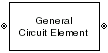# General Circuit Element

Model two-port network described by `rfckt` object

• Library:
• RF Blockset / Equivalent Baseband / Black Box Elements

•## Description

The General Circuit Element block models the two-port network described by an RF Toolbox™ circuit (`rfckt`) object. The block uses the `rfckt/analyze` method to calculate the network parameters at the modeling frequencies.

To learn how you can use General Circuit Elementblock to realize cascaded filters, see Multiple Realizations of Cascaded Filters example.

## Parameters

expand all

### Main

RFCKT object that contains circuit element device data, specified as an any RF Toolbox rfckt object that creates a default circuit object of the specified type, or a MATLAB® expression that generates such an object. See RF Circuit Objects for more information about circuit objects.

### Visualization

Frequency data source, specified as `User-specified`.

Frequency data range, specified as a vector with each element unit in hertz.

Reference impedance, specified as a nonnegative scalar in ohms.

Type of data plot to visualize using the given data, specified as one of the following:

• `X-Y plane` — Generate a Cartesian plot of the data versus frequency. To create linear, semilog, or log-log plots, set the Y-axis scale and X-axis scale accordingly.

• `Composite data` — Plot the composite data. For more information, see Create Plots.

• `Polar plane` — Generate a polar plot of the data. The block plots only the range of data corresponding to the specified frequencies.

• `Z smith chart`, ```Y smith chart```, and `ZY smith chart` — Generate a Smith® chart. The block plots only the range of data corresponding to the specified frequencies.

Type of parameters to plot, specified as one of the following:

 `S11` `S12` `S21` `S22` `GroupDelay` `GammaIn` `GammaOut` `VSWRIn` `VSWROut` `OIP3` `IIP3` `NF` `NFactor` `NTemp` `TF1` `TF2` `TF3` `Gt` `Ga` `Gp` `Gmag` `Gmsg` `GammaMS` `GammaML` `K` `Delta` `Mu` `MuPrime`

Note

Y parameter1 is disabled when you select Plot type to `Composite data`.

Type of parameters to plot, specified as one of the following:

 `S11` `S12` `S21` `S22` `GroupDelay` `GammaIn` `GammaOut` `VSWRIn` `VSWROut` `OIP3` `IIP3` `NF` `NFactor` `NTemp` `TF1` `TF2` `TF3` `Gt` `Ga` `Gp` `Gmag` `Gmsg` `GammaMS` `GammaML` `K` `Delta` `Mu` `MuPrime`

Note

Y parameter2 is disabled when you select Plot type to `Composite data`.

Plot format, specified as one of the following.

Y parameter1Y format1
`S11`, `S12`, `S21`, `S22`, `GammaIn`, `GammaOut`, `TF1`, `TF2`, `TF3`, `GammaMS`, `GammaML`, and `Delta`.`dB`, ```Magnitude (decibels)```, `Abs`, `Mag`, ```Magnitude (linear)```, `Angle`, `Angle(degrees)`, `Angle(radians)`, `Real`, `Imag`, and `Imaginary`.
`GroupDelay``ns`, `us`, `ms`, `s`, and `ps`.
`VSWRIn` and `VSWROut`.`Magnitude (decibels)` and `None`.
`OIP3` and `IIP3`.`dBm`, `W`, and `mW`.
`NF` `dB` and ```Magnitude (decibels)```.
`NFactor`, `K`, `Mu`, and `MuPrime`.`None`
`NTemp``Kelvin`
`Gt`, `Ga`, `Gp`, `Gmag`, and `Gmsg`.`dB`, ```Magnitude (decibels)```, and `None`.

#### Dependencies

To enable Y format1, set Plot type to `X-Y plane`.

Plot format, specified as one of the following.

Y parameter2Y format2
`S11`, `S12`, `S21`, `S22`, `GammaIn`, `GammaOut`, `TF1`, `TF2`, `TF3`, `GammaMS`, `GammaML`, and `Delta`.`dB`, ```Magnitude (decibels)```, `Abs`, `Mag`, ```Magnitude (linear)```, `Angle`, `Angle(degrees)`, `Angle(radians)`, `Real`, `Imag`, and `Imaginary`.
`GroupDelay``ns`, `us`, `ms`, `s`, and `ps`.
`VSWRIn` and `VSWROut`.`Magnitude (decibels)` and `None`.
`OIP3` and `IIP3`.`dBm`, `W`, and `mW`.
`NF` `dB` and ```Magnitude (decibels)```.
`NFactor`, `K`, `Mu`, and `MuPrime`.`None`
`NTemp``Kelvin`
`Gt`, `Ga`, `Gp`, `Gmag`, and `Gmsg`.`dB`, ```Magnitude (decibels)```, and `None`.

#### Dependencies

To enable Y format2, set Plot type to `X-Y plane`.

Frequency plot, specified as `Freq`.

Frequency plot format, specified as one of the following.

 `Auto` `Hz` `kHz` `MHz` `GHz` `THz`

Y-axis scale, specified as `Linear` or `Log`.

#### Dependencies

To enable this parameter, set Plot type to ```X-Y plane```.

X-axis scale, specified as `Linear` or `Log`.

#### Dependencies

To enable this parameter, set Plot type to ```X-Y plane```.

Plot specified data using the plot button.